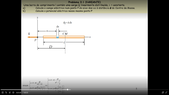# Calculating eletric potential using line integral of electric field

• pedromatias

#### pedromatias

Homework Statement
A bar of length l has charge Q that is linearly distributed, with constant charge density λ. There is a point P at distance D from the center of mass of the bar, and P is in the same axis as the bar. Knowing the electric field, calculate the electric potential at P using a line integral. See figure.
Relevant Equations
The difference of potential between two points is symmetric to the line integral between those two points of the dot product between the electric field and the line.
So, I am able to calculate the electric potential in another way but I know that this way is supposed to work as well, but I don't get the correct result.

I calculated the electric field at P in the previous exercise and its absolute value is $$E = \frac {k Q} {D^2-0.25*l^2}$$ This is correct as per the solution. From the figure, we can see that the direction of the electric field at P is that of the negative x direction.

So, to calculate the electric potential I took advantage of the fact that at infinite the potential is zero and did

$$V_P = \int_D^{\infty} \frac {k Q} {r^2-0.25*l^2} \, dr$$

However, this integral does not give the correct result. I inserted it into integral calculator and the result was not the correct one, which is

$$k \lambda \ln \frac {D+\frac{l}{2}} {D-\frac{l}{2}}$$

I don't understand what I am doing incorrectly. My reasoning is that I have the expression for the Electric field in a point at a distance ##r## in the axis of ##P##, and that Electric field points in the same direction as the straight line path from ##P## to ##\infty##, so the dot product is the magnitude of the electric field.

The way I solved it correctly and which gives me the right solution is, where ##x## is the distance from P,

$$dv = k \frac {dq}{x}$$
$$dv = k \lambda \frac {dx}{x}$$
$$V = \int_{D-0.5l}^{D+0.5l} \frac {k \lambda} {x} \, dx$$
$$V = k \lambda \ln \frac {D+\frac{l}{2}} {D-\frac{l}{2}}$$

#### Attachments

•Screenshot (130).png
15.3 KB · Views: 45
What result does your calculator give? How have you verified that it is different from the one you got? Sometimes solutions given by calculators look different but aren't.

•pedromatias and vela